Courses

Test: Mode Of Grouped Data

10 Questions MCQ Test Mathematics (Maths) Class 10 | Test: Mode Of Grouped Data

Description
Attempt Test: Mode Of Grouped Data | 10 questions in 10 minutes | Mock test for Class 10 preparation | Free important questions MCQ to study Mathematics (Maths) Class 10 for Class 10 Exam | Download free PDF with solutions
QUESTION: 1

The modal class in the following frequency distribution is: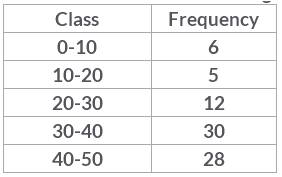Solution:

The modal class is the class with the highest frequency ie, the class which occurs the most. So,30-40 has frequency 30 which is the highest, therefore, 30-40 is the modal class.

QUESTION: 2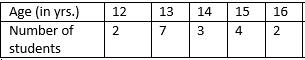Modal age of students in given distribution is​

Solution:

Mode is the most frequently occurring observation. Since it's not a grouped data the frequency is the occurrence of only one observation , So the highest no. of frequency is 7 . So the modal age is 13.

QUESTION: 3

Modal class of the following distribution is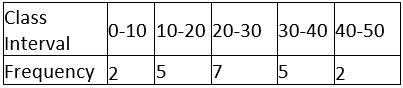​

Solution:
QUESTION: 4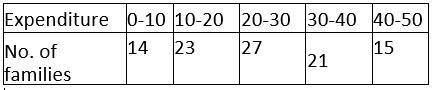What is the mode of the given data?​

Solution:

Mode=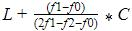Where L=lower limit of the modal class =20
f1= modal class frequency.=27
f2 = just after the modal class frequency.=21
f0 = just previous the modal class frequency=23
C = Class size=10
Mode=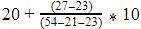=20+4=24

QUESTION: 5

The age of 18 students of a class is reported below. Their modal age is 10, 17, 14, 10, 11, 12, 12, 13, 17, 13, 14, 14, 15, 16, 17, 15, 17, 16​

Solution:

Mode is the highest number of times an observation occurs, which means the number that appears most frequently in a set. So modal age is the age which occurs maximum number of times. It is evident that 17 occurs most of the times. So, modal age is 17 years.

QUESTION: 6

The lower limit of the modal class of the following data is: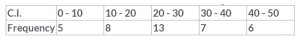Solution:

Modal class is the interval which has the highest frequency ie, which occur most of the times.Here highest frequency is 13 in the class interval 20-30. So lower limit of the class interval is 20.

QUESTION: 7

Find out the mode of the following data: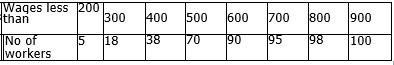Solution:

The mode of a set of data values is the value that appears most often .So mode is the most occurrence of observation.
Converting the distribution in continuous grouped and applying the formula
Mode=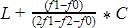Modal class is 400-500
Where L=lower limit of the modal class =400
f1= modal class frequency=32
f2 = just after the modal class frequency=20
f0 = just previous the modal class frequency=20
C = Class size=100
Mode=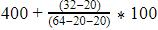=400+50=450

QUESTION: 8

Mode is not affected by

Solution:

The mode of a set of data values is the value that appears most often.it is the value that is most likely to be sampled This means mode is the observations whose frequency is highest. So it does not pay attention to what is the maximum value or the minimum value . So it is not affected either by maximum or minimum values and its not affected by both as well.

QUESTION: 9

For the following distribution the modal class is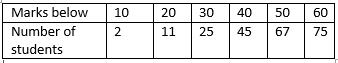Solution:
QUESTION: 10

The mode of 12, 17, 16, 14, 13, 16, 11, 14 is

Solution:Use Code STAYHOME200 and get INR 200 additional OFF Use Coupon Code

Track your progress, build streaks, highlight & save important lessons and more!

Similar ContentRelated tests